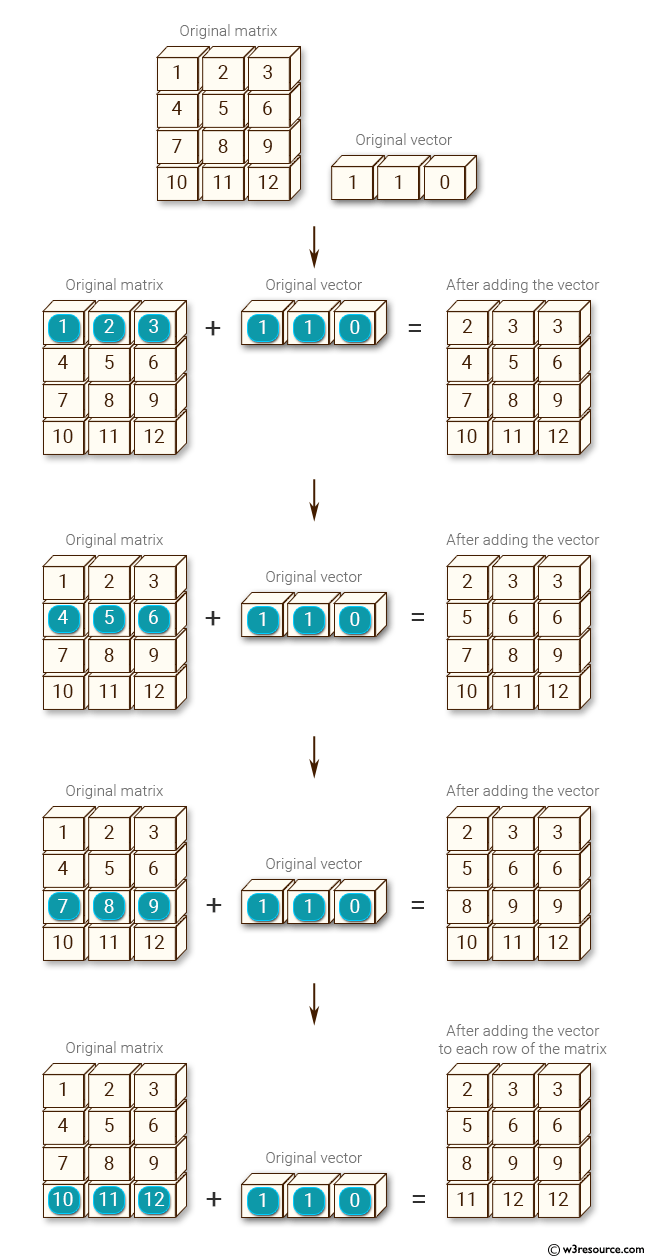﻿ NumPy: Add a vector to each row of a given matrix - w3resource# NumPy: Add a vector to each row of a given matrix

## NumPy: Basic Exercise-34 with Solution

Write a NumPy program to add a vector to each row of a given matrix.

Sample Solution :

Python Code :

``````import numpy as np
m = np.array([[1,2,3], [4,5,6], [7,8,9], [10, 11, 12]])
v = np.array([1, 1, 0])
print("Original vector:")
print(v)
print("Original matrix:")
print(m)
result = np.empty_like(m)
for i in range(4):
result[i, :] = m[i, :] + v
print("\nAfter adding the vector v to each row of the matrix m:")
print(result)
``````

Sample Output:

```Original vector:
[1 1 0]
Original matrix:
[[ 1  2  3]
[ 4  5  6]
[ 7  8  9]
[10 11 12]]

After adding the vector v to each row of the matrix m:
[[ 2  3  3]
[ 5  6  6]
[ 8  9  9]
[11 12 12]]
```

Pictorial Presentation:Python Code Editor:

Have another way to solve this solution? Contribute your code (and comments) through Disqus.

What is the difficulty level of this exercise?

Test your Python skills with w3resource's quiz

﻿

## Python: Tips of the Day

Checks if the given number falls within the given range.

Example:

```def tips_range(n, start, end = 0):
return start <= n <= end if end >= start else end <= n <= start
print(tips_range(2, 4, 6))
print(tips_range(4, 8))
print(tips_range(1, 3, 5))
print(tips_range(1, 3))
```

Output:

```False
True
False
True
```